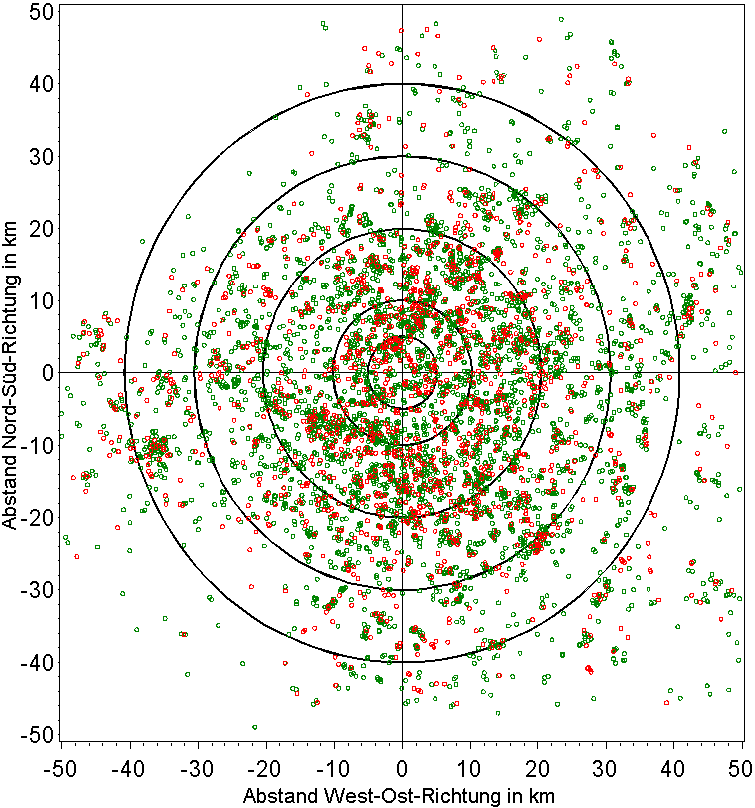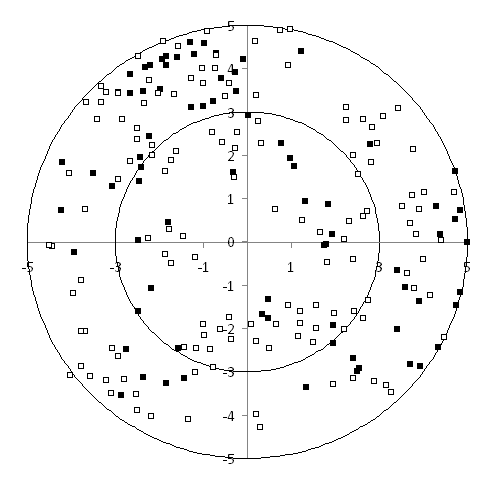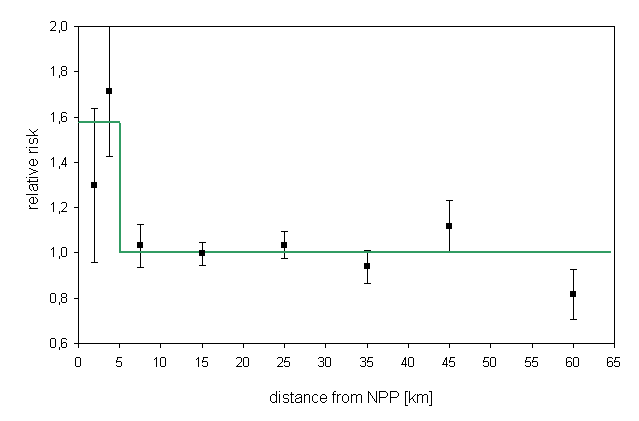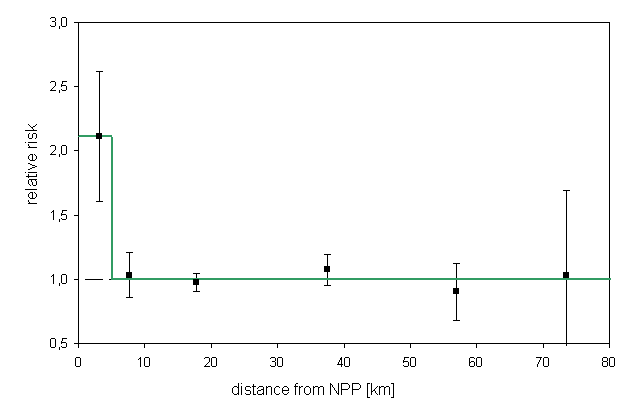The German KiKK study

In 2002 the German Radiation Protection Agency (Bundesamt für Strahlenschutz, BfS) commissioned a case-control study to investigate childhood cancers near nuclear power plants. This so called KiKK study (Kinderkrebs um Kernkraftwerke) was conducted by the German Childhood Registry (Deutsches Kinderkrebsregister, DKKR) in Mainz.
The results of the KiKK study were presented in a technical report in December 2007. At the same time, the results were published in two scientific journals. The articles can be accessed in the web (see below).

The polar diagram below (Fig.3.3 in KiKK study part 1) shows cancer cases (red) und controls (green) within 50 km distance from the nearest NPP site.The KiKK report shows the following table (Table 3.14. part 1) containing the numbers of cases (Fälle, F) and controls (Kontrollen, K) in 7 distance categories.

 r F K 0-5 km 77 148 5-10 km 158 464 10-20 km 523 1589 20-30 km 403 1181 30-40 km 225 726 40-50 km 137 371 > 50 km 69 256 Summe 1592 4735

The 5-km range in Figure 1 can be studied in more detail.  Using a graphics program it was possible to determine the number of cases (N=21, Fig. bottom, black dots) and controls (N=49, white dots) within the 3-km range. The number of cases and controls in the 3-5 km range are then obtained by substraction from the published numbers for the whole 5-km area.A logistic regression with model

ln(F/K)=beta0+beta1/r

yields the following results for the parameters beta0. beta1:

 parameter estimate SE z-value p-value beta0 -1,1581 0,0406 -28,534 <0,0001 beta1 1,0477 0,4309 2,431 0,0150

SE: Standard error of estimate
z-value: estimate divided by SE
p-value: two-sided p-value

The result for beta1 agrees with the result in Table 3.15 of the KiKK study (1,18 +- 0,44; p=0,0034).

A better fit to the data is obtained with the following categorial regression model

ln(F/K)=beta0+beta1*d5km,

where d5km is a dummy variable for the 5-km region (d5km=1 for r<5km und d5km=0 for r>5km).

Die following Figure shows the relative risks (= odds ratios) in the individual distance zones and the regression result.Leukemia

KiKK part 1 also contains results for leukemia cases. The numbers of cases (F) and controls (K), together with the harmonic means of the distances (r), are found in the article by Kaatsch et al. for 6 distance zones.

 r F K 3.09 37 54 7.62 58 173 17.79 332 1048 37.45 135 387 56.98 27 92 73.59 4 12 Summe 593 1766

Regressions with the following 5 models are conducted:

Model 1: ln(F/K) = beta0 + beta1/r
Model 2: ln(F/K) = beta0 + beta1/r + beta2/r^2
Model 3: ln(F/K) = beta0 + beta1/r^2
Model 4: ln(F/K) = beta0 + beta1/r + beta2*d5km
Model 5: ln(F/K) = beta0 + beta1*d5km

dummy-variable d5km is 1 for r < 5 km and 0 for r > 5 km

Model information:

 DF SSE AIC model 1 4 3.3148 39.538 model 2 3 0.7197 38.943 model 3 4 1.2925 37.516 model 4 3 0.9244 39.148 model 5 4 0.9260 37.149

DF = degrees of freedom
SSE = sum of squares (deviance)
AIC = AIC criterion of the goodness of fit

The following table shows the parameter estimates and standard errors (SE) resulting from the 5 regressions:

 Parameter estimate SE z value P value Model 1 beta0 -1.2393 0.0695 -17.84 0.0000 beta1 2.2113 0.7387 2.994 0.0028 Model 2 beta0 -1.0600 0.1310 -8.092 0.0000 beta1 -2.1110 2.7950 -0.755 0.4501 beta2 13.061 8.1320 1.606 0.1082 Model 3 beta0 -1.1511 0.0512 -22.469 0.0000 beta1 7.1316 2.1303 3.348 0.0008 Model 4 beta0 -1.1210 0.1032 -10.858 0.0000 beta1 -0.0663 1.6631 -0.040 0.9682 beta2 0.7644 0.4980 1.535 0.1248 Model 5 beta0 -1.1247 0.0488 -23.041 0.0000 beta1 0.7466 0.2189 3.410 0.0006

Results:

• Model 1 yields a significant regression coefficient beta1 (p=0.0028)

• Model 2 (linear-quadratic model) yields a better fit than model 1. The sum of squares (SSE) is 0.720 which compares to 3.315 for the linear model, the corresponding values of AIC are 38.94 and 39.54. The estimate for the linear term is negative.

• Model 3 (quadratic distance trend) fits the data  better than the linear model (SSE=1.293, AIC=37.52).

• Model 4 which allows for excess in the 5-km zone yields a negative regression coefficient beta1. This means that for r > 5 km the risk does not decrease with increasing distance from the NPP.

• Model 5 which only allows for an excess in the 5-km zone yields the best fit to the data by the AIC criterion (AIC=37.15)

• The RRs obtained with the linear model translate to 81 excess cases in the study zone (90% CI: 39-118), and to 34 excess cases (90% VB: 19 bis 47) with the quadratic model.

The following figure shows the relative risk (odds ratio) as a function of distance from the NPP and the regression line with the categorial model (model 5).References

Spix C. Schmiedel S. Kaatsch P. Schulze-Rath R. Blettner M. Case-control study on childhood cancer in the vicinity of nuclear power plants in Germany 1980-2003. Eur J Cancer. 2008 Jan;44(2):275-84. Abstract